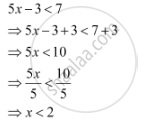CBSE (Arts) Class 11CBSE
Share

# Solve 5x– 3 < 7, When X Is an Integer - CBSE (Arts) Class 11 - Mathematics

ConceptAlgebraic Solutions of Linear Inequalities in One Variable and Their Graphical Representation

#### Question

Solve 5x– 3 < 7, when x is an integer

#### Solution

The given inequality is 5x– 3 < 7.The integers less than 2 are …, –4, –3, –2, –1, 0, 1.

Thus, when x is an integer, the solutions of the given inequality are

…, –4, –3, –2, –1, 0, 1.

Hence, in this case, the solution set is {…, –4, –3, –2, –1, 0, 1}.

Is there an error in this question or solution?

#### APPEARS IN

NCERT Solution for Mathematics Textbook for Class 11 (2018 to Current)
Chapter 6: Linear Inequalities
Q: 3.1 | Page no. 122
Solution Solve 5x– 3 < 7, When X Is an Integer Concept: Algebraic Solutions of Linear Inequalities in One Variable and Their Graphical Representation.
S